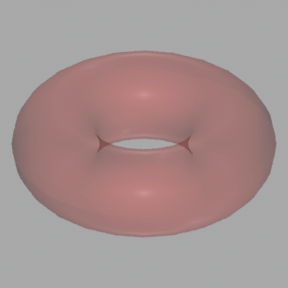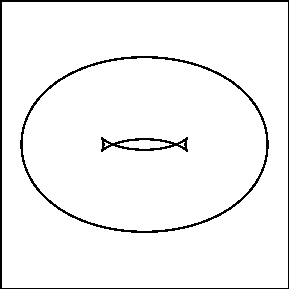# Projections, Profiles, and Envelopes

## "Elimination = Projection"

Suppose we are given a system of equations, for example

y-x2=0
z-x3=0

that defines a curve in (x,y,z) 3-space called the twisted cubic curve, whose projection onto the yz-plane is shown below. You can click on the graph of the projection to generate an MPEG movie (308K) of the curve in 3-space.

If we try to eliminate the variable x from those equations, we can produce the equation z2-y3=0, which is the curve with a cusp in the yz-plane:

### The curve z2 - y3=0.

If you project the twisted cubic curve orthogonally onto the yz-plane, this curve with a cusp is exactly what you hit. It should at least be clear that the equation z2-y3 produced by elimination of the variable x is satisfied by all points in the projection, since the projection map takes a point (x,y,z) satisfying the first two equations (and hence also satisfying z2-y3) and maps it to (y,z).

## Questions to think about

What equation will describe the projection of the twisted cubic onto the xy-plane? What about onto the xz-plane?
More generally, if we are given a system of equations

f1(x1,...,xn)=0
f2(x1,...,xn)=0
etc.

they define a subset V of the n-space with coordinates x1,..., xn, Then any equations g(xk,xk+1,...,xn)=0 obtained from the fi's by elimination of the variables x1,...,xk-1 will be satisfied by all points in the projection of V onto the (n-k+1)-subspace with coordinates xk,xk+1,...,xn. This is part of what we mean by "elimination = projection". For a more detailed discussion of the case where the fi are polynomials, and a more precise statement about the relation between elimination and projection in this case, see Cox, Little, and O'Shea Sections 3.1 and 3.2.

## Profiles of Surfaces

Suppose we are looking at a surface S in 3-space with coordinates (x,y,z), and our viewpoint is from above on the z-axis. For example, take the tilted torus shown below. If we were to draw a 2-dimensional line drawing of the torus projected onto the xy-plane, certain points of the torus will show up in this silhouette (or shadow or profile) while others will not:### The tilted torus and its shadow on the xy-plane

How did we decide which points (x,y,z) on the torus to project into the plane? If you stare at the picture and think about it, you'll see that we chose to project the points where the tangent plane to the torus is parallel to the z-axis. We will call this set the z-profile of the surface.

To find equations in x,y that contain the z-profile, we must eliminate the variable z from the equations in x,y,z that define points on the torus that have tangent plane parallel to the z-axis. So first we need to figure out what those equations are.

## Questions to think about

• Explain how to calculate the normal vector at a point P on the surface f(x,y,z)=0 using facts about tangent planes and normals to level sets of a function that you learned in multivariable calculus. (Hint: the gradient vector (df/dx, df/dy, df/dz) of f evaluated at P is involved somehow).
• Once you have the normal vector at P, how can you describe the condition that the tangent plane is parallel to the z-axis?
• Put this together to explain why the points in the z-profile should be the projections to the xy-plane of the solutions (x,y,z) of
f(x,y,z)=0
d/dz f(x,y,z)=0

This suggests that if f(x,y,z) is a polynomial, we could compute the equation for the z-profile of the surface f(x,y,z)=0 using a Gröbner basis computation in Maple to eliminate variables from the two equations above. This is exactly what we did for the tilted torus.

## Envelope of a Family of Curves

If we are given a family of curves Ct defined by F(t,x,y)=0 in the xy-plane, a curve which has a common tangent with each one of curves Ct is said to be part of the envelope of Ct. For example, if Ct is the family of circles of radius t3/3 centered about the point (t2,t3/3) for various values of t, pictured below, with part of its envelope highlighted:## Questions to think about

What is the equation F(t,x,y) = 0 defining Ct in this case? Try to write down a polynomial equation, i.e. one that avoids square roots.
We would like to be able to compute an equation satisfied by the points of the envelope if we are given the equation F(t,x,y)=0. The key observation is this: the envelope is a subset of the t-profile of the surface defined by F(t,x,y)=0 in (t,x,y)-space, as shown below. Click on the graphic to generate an MPEG movie (410 Kbytes) of this surface.

### The surface F(t,x,y)=0 produced by the family of circles

This then suggests that when F(t,x,y) is a polynomial, we can follow the recipe for computing the t-profile of the surface F(t,x,y) to find the equations satisfied by the points of the envelope. That is, we eliminate t (using a Gröbner basis computation) from the equations

F(t,x,y)=0
d/dt F(t,x,y)=0

Here is the computation for the family of circles we discussed earlier:

```>F:=(x-t^2)^2+(y-t^3/3)^2-(t^3/3)^2;

2 2             3 2        6
F := (x - t )  + (y - 1/3 t )  - 1/9 t

> GB:=gbasis({F,diff(F,t)},[t,x,y],plex);
```
Only the 7th (last) polynomial in the resulting Gröbner basis did not involve t, so by factoring it we obtain the equations for the envelope curves:
```> factor(GB);

2    2       3      2  2       2          2      4
y (x  + y ) (64 x  + 3 y  x  + 72 y  x - 144 y  + 3 y )

```
The resulting implicit equation for the envelope can then be plotted to obtain the red curves in the 4th figure on this page.

## Questions to think about

• Can you explain why the ``extra" factor y shows up in the envelope equation? What does it correspond to in the picture of the envelope?
• Does the extra factor x2+y2 in the envelope equation correspond to something you can see in the picture?

For a different (and more detailed) discussion of envelopes, see Cox, Little, and O'Shea Section 3.4. For an even deeper discussion, see Bruce and Giblin.
Go To: Computing the Envelope of a Family of Curves
Go To: Gröbner bases and Elimination of Variables
Up: Introduction
Vic Reiner <reiner@math.umn.edu>
Frederick J. Wicklin <fjw@geom.umn.edu>
Last modified: Thu Apr 25 08:20:55 1996